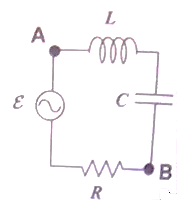Problem: In the circuit shown below, the AC generator supplies an EMF of the form ε = 15sin(100t - π/3) volts. A student measures the current to be I = 6 sin(100t) amps. Thus the generator voltage lags the current by π/3 radian (i.e. 60°). The resistance R must be:a. 1.25 Ωb. 2.5 Ωc. 5.0 Ωd. 7.5 Ωe. cannot be determined from the information given.

FREE Expert Solution
94% (276 ratings)
Problem Details

In the circuit shown below, the AC generator supplies an EMF of the form ε = 15sin(100t - π/3) volts. A student measures the current to be I = 6 sin(100t) amps. Thus the generator voltage lags the current by π/3 radian (i.e. 60°). The resistance R must be:

a. 1.25 Ω

b. 2.5 Ω

c. 5.0 Ω

d. 7.5 Ω

e. cannot be determined from the information given.